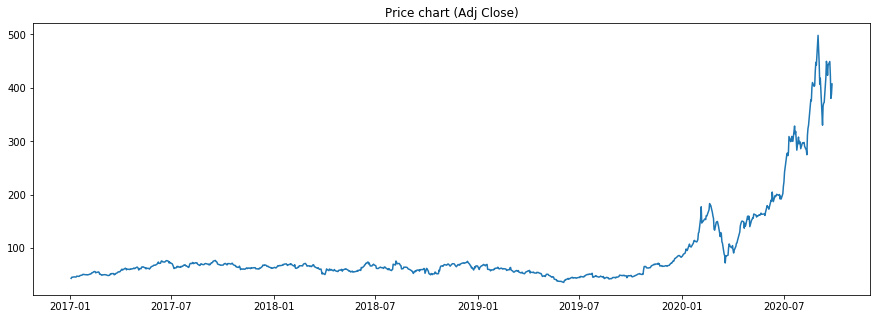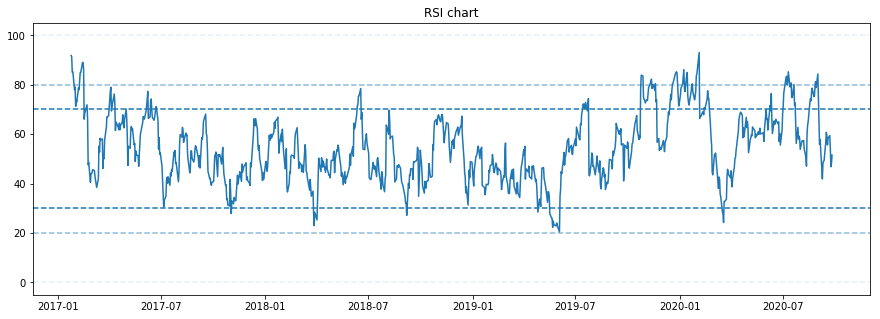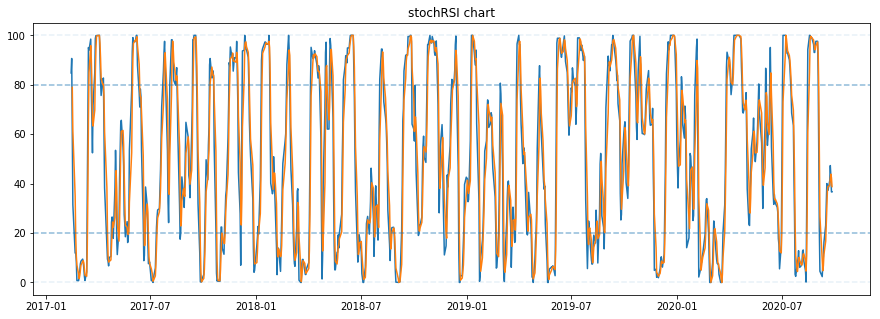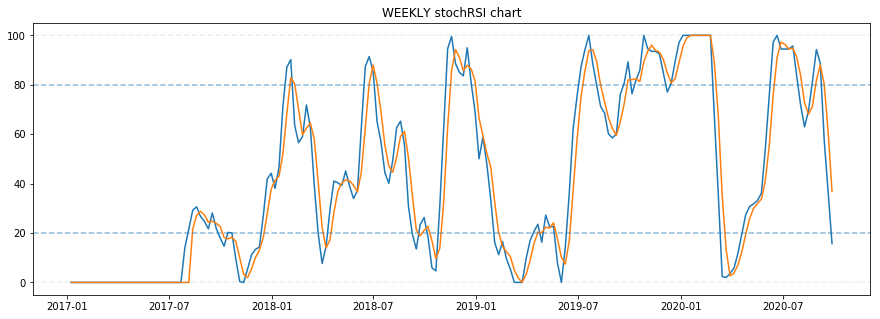Stochastic RSI computation with Python¶

Stochastic RSI indicator is derived from the values of regular RSI indicator (Relative Strength Index). It means it indicates changes in another indicator. This can cause to create lots of false trading signals.

First we will need to compute ragular RSI, detailed proces is described here:
https://tcoil.info/compute-rsi-for-stocks-with-python-relative-strength-index/

Once we have RSI, we can proceed to compute the Stochastic RSI.

Equation:

$$StochRSI(n)_{i} = \frac{RSI(n)_{i} - minRSI(n)_{i}}{maxRSI(n)_{i} - minRSI(n)_{i}} \times 100$$

$StochRSI(n)_{i}$ ... Stochastic RSI of window n at step i
$RSI(n)_{i}$ ... RSI of window n at step i
$minRSI(n)_{i}$ ... lowest RSI value looking back over n datapoint window at step i
$maxRSI(n)_{i}$ ... highest RSI value looking back over n datapoint window at step i

We took the equation from Fidelity, this gives us the value range between 0 and 100: https://www.fidelity.com/learning-center/trading-investing/technical-analysis/technical-indicator-guide/stochrsi

But we are not finished yet. This equation will give us only one datapoint line. We would like to have the same graphs as on tradingview.com for Stochastic RSI.

For that we will check definition of regular Stochastic oscillator and compare it to the equation above.

$$\%K(n)_{i} = \frac{Close_{i} - minClose(n)_{i}}{maxClose(n)_{i} - minClose(n)_{i}} \times 100$$

$\%K(n)_{i}$ ... so called Slow stochastic indicator at step i
$Close(n)_{i}$ ... RSI of window n at step i
$minClose(n)_{i}$ ... lowest closing price looking back over n datapoint window at step i
$maxClose(n)_{i}$ ... highest closing looking back over n datapoint window at step i
$\%D(n)_{i}$ ... so called Fast stochastic indicator at step i, it is typically 3 datapoint moving average of $\%K$

From that we see that if we feed RSI values into Stochastic equation, we get ...
... wait for it ...
Yes, we get Stochastic RSI.

We will construct the graph using $\%K$ and $\%D$.

Buy/Sell signals are generated when $\%K$ and $\%D$ lines cross (and ideally when they cross when stochastic is in oversold or overbought levels)

Oversold level: values below or equal 20 Overbought level: above or equal 80

Regular Stochastic definition: https://www.investopedia.com/terms/s/stochasticoscillator.asp

Tradingview is showing parameters for the stiochastic RSI function like this:
StochRSI(3,3,14,14)

which suggests that we take RSI 14 window, then from that we take Stochastic 14 window and then both %K and %D are smoothed with 3 window. Smoothing for %K was not defined in above equations but I will implement it in the code, because I take tradingview as a benchmark.

Importing libraries¶

In :
#optional installations:

from IPython.core.display import display, HTML
display(HTML("<style>.container { width:100% !important; }</style>"))

# ___library_import_statements___
import pandas as pd

# for pandas_datareader, otherwise it might have issues, sometimes there is some version mismatch
pd.core.common.is_list_like = pd.api.types.is_list_like

# make pandas to print dataframes nicely
pd.set_option('expand_frame_repr', False)

import numpy as np
import matplotlib.pyplot as plt
import datetime
import time

import yfinance as yahoo_finance

#optional
#yahoo_finance.pdr_override()

%matplotlib inline
In :
# ___variables___
ticker = 'TSLA'

start_time = datetime.datetime(2017, 1, 1)
#end_time = datetime.datetime(2019, 1, 20)
end_time = datetime.datetime.now().date().isoformat()         # today

Get stock data¶

In :
# yahoo gives only daily historical data
connected = False
while not connected:
try:
ticker_df = web.get_data_yahoo(ticker, start=start_time, end=end_time)
connected = True
print('connected to yahoo')
except Exception as e:
print("type error: " + str(e))
time.sleep( 5 )
pass

# use numerical integer index instead of date
#ticker_df = ticker_df.reset_index()
connected to yahoo
High        Low       Open      Close      Volume  Adj Close
Date
2017-01-03  44.066002  42.192001  42.972000  43.397999  29616500.0  43.397999
2017-01-04  45.599998  42.862000  42.950001  45.397999  56067500.0  45.397999
2017-01-05  45.495998  44.389999  45.284000  45.349998  29558500.0  45.349998
2017-01-06  46.062000  45.090000  45.386002  45.801998  27639500.0  45.801998
2017-01-09  46.383999  45.599998  45.793999  46.256001  19897500.0  46.256001
In :
df = ticker_df

Compute RSI¶

In :
def computeRSI (data, time_window):
diff = data.diff(1).dropna()        # diff in one field(one day)

#this preservers dimensions off diff values
up_chg = 0 * diff
down_chg = 0 * diff

# up change is equal to the positive difference, otherwise equal to zero
up_chg[diff > 0] = diff[ diff>0 ]

# down change is equal to negative deifference, otherwise equal to zero
down_chg[diff < 0] = diff[ diff < 0 ]

# check pandas documentation for ewm
# https://pandas.pydata.org/pandas-docs/stable/reference/api/pandas.DataFrame.ewm.html
# values are related to exponential decay
# we set com=time_window-1 so we get decay alpha=1/time_window
up_chg_avg   = up_chg.ewm(com=time_window-1 , min_periods=time_window).mean()
down_chg_avg = down_chg.ewm(com=time_window-1 , min_periods=time_window).mean()

rs = abs(up_chg_avg/down_chg_avg)
rsi = 100 - 100/(1+rs)
return rsi
In :
In :
print(df.tail())
High        Low       Open      Close      Volume  Adj Close  RSI
Date
2017-01-03  44.066002  42.192001  42.972000  43.397999  29616500.0  43.397999  NaN
2017-01-04  45.599998  42.862000  42.950001  45.397999  56067500.0  45.397999  NaN
2017-01-05  45.495998  44.389999  45.284000  45.349998  29558500.0  45.349998  NaN
2017-01-06  46.062000  45.090000  45.386002  45.801998  27639500.0  45.801998  NaN
2017-01-09  46.383999  45.599998  45.793999  46.256001  19897500.0  46.256001  NaN
High         Low        Open       Close       Volume   Adj Close        RSI
Date
2020-09-21  455.679993  407.070007  453.130005  449.390015  109476800.0  449.390015  59.430364
2020-09-22  437.760010  417.600006  429.600006  424.230011   79580800.0  424.230011  54.334777
2020-09-23  412.149994  375.880005  405.160004  380.359985   95074200.0  380.359985  46.799953
2020-09-24  399.500000  351.299988  363.799988  387.790009   96561100.0  387.790009  48.112353
2020-09-25  408.730011  391.299988  393.470001  407.339996   67068400.0  407.339996  51.502471

Stochastic¶

In :
def stochastic(data, k_window, d_window, window):

# input to function is one column from df
# containing closing price or whatever value we want to extract K and D from

min_val  = data.rolling(window=window, center=False).min()
max_val = data.rolling(window=window, center=False).max()

stoch = ( (data - min_val) / (max_val - min_val) ) * 100

K = stoch.rolling(window=k_window, center=False).mean()
#K = stoch

D = K.rolling(window=d_window, center=False).mean()

return K, D
In :
df['K'], df['D'] = stochastic(df['RSI'], 3, 3, 14)
In :
Out:
High Low Open Close Volume Adj Close RSI K D
Date
2017-01-03 44.066002 42.192001 42.972000 43.397999 29616500.0 43.397999 NaN NaN NaN
2017-01-04 45.599998 42.862000 42.950001 45.397999 56067500.0 45.397999 NaN NaN NaN
2017-01-05 45.495998 44.389999 45.284000 45.349998 29558500.0 45.349998 NaN NaN NaN
2017-01-06 46.062000 45.090000 45.386002 45.801998 27639500.0 45.801998 NaN NaN NaN
2017-01-09 46.383999 45.599998 45.793999 46.256001 19897500.0 46.256001 NaN NaN NaN
In :
df.tail()
Out:
High Low Open Close Volume Adj Close RSI K D
Date
2020-09-21 455.679993 407.070007 453.130005 449.390015 109476800.0 449.390015 59.430364 41.170330 39.213510
2020-09-22 437.760010 417.600006 429.600006 424.230011 79580800.0 424.230011 54.334777 47.305336 41.927382
2020-09-23 412.149994 375.880005 405.160004 380.359985 95074200.0 380.359985 46.799953 43.049589 43.841752
2020-09-24 399.500000 351.299988 363.799988 387.790009 96561100.0 387.790009 48.112353 36.618415 42.324447
2020-09-25 408.730011 391.299988 393.470001 407.339996 67068400.0 407.339996 51.502471 36.678445 38.782150

Plotting¶

In :
def plot_price(df):
# plot price
plt.figure(figsize=(15,5))
plt.show()
return None

def plot_RSI(df):
# plot correspondingRSI values and significant levels
plt.figure(figsize=(15,5))
plt.title('RSI chart')
plt.plot(df['RSI'])

plt.axhline(0, linestyle='--', alpha=0.1)
plt.axhline(20, linestyle='--', alpha=0.5)
plt.axhline(30, linestyle='--')

plt.axhline(70, linestyle='--')
plt.axhline(80, linestyle='--', alpha=0.5)
plt.axhline(100, linestyle='--', alpha=0.1)
plt.show()
return None

def plot_stoch_RSI(df):
# plot corresponding Stoch RSI values and significant levels
plt.figure(figsize=(15,5))
plt.title('stochRSI chart')
plt.plot(df['K'])
plt.plot(df['D'])

plt.axhline(0, linestyle='--', alpha=0.1)
plt.axhline(20, linestyle='--', alpha=0.5)
#plt.axhline(30, linestyle='--')

#plt.axhline(70, linestyle='--')
plt.axhline(80, linestyle='--', alpha=0.5)
plt.axhline(100, linestyle='--', alpha=0.1)
plt.show()
return None

def plot_all(df):
plot_price(df)
plot_RSI(df)
plot_stoch_RSI(df)
return None
In :
plot_all(df)As we can see, the stochastic RSI is quite noisy. Either we can trade on shorter timeframes or if we prefer longer term swing trading we can analyze weekly Stochastic RSI that should give much stronger signals.

Supressing false signals in stoch RSI¶

We can supress the noise by using weekly timeframe. Note that by using weekly timeframe, you will lose lots of trailing data, since we are using 14 week window RSI and from that we have 14 week window stochastic.

In :
# weekly timeframe aggregation

agg_dict = {'Open': 'first',
'High': 'max',
'Low': 'min',
'Close': 'last',
'Volume': 'mean'}

# resampled dataframe
# 'W' means weekly aggregation
df_res = df.resample('W').agg(agg_dict)
In :
In :
df_res['K'], df_res['D'] = stochastic(df_res['RSI'], 3, 3, 14)
In :
def plot_weekly_stoch_RSI(df_res):
# WEEKLY plot corresponding Stoch RSI values and significant levels
plt.figure(figsize=(15,5))
plt.title('WEEKLY stochRSI chart')

df_res = df_res.reset_index()
plt.plot(df_res['Date'], df_res['K'].fillna(0))   # NaN to zeros so plot is in scale
plt.plot(df_res['Date'], df_res['D'].fillna(0))   # NaN to zeros so plot is in scale

#df_res.reset_index().plot(x='Date', y='K', figsize=(15,5))
#df_res.reset_index().plot(x='Date', y='D', figsize=(15,5))

plt.axhline(0, linestyle='--', alpha=0.1)
plt.axhline(20, linestyle='--', alpha=0.5)
#plt.axhline(30, linestyle='--')

#plt.axhline(70, linestyle='--')
plt.axhline(80, linestyle='--', alpha=0.5)
plt.axhline(100, linestyle='--', alpha=0.1)
plt.show()
return None

def plot_mixed(df, df_res):
plot_price(df)
plot_RSI(df)
plot_weekly_stoch_RSI(df_res)
return None
In :
plot_mixed(df, df_res)The weekly stochastic signals look pretty actionable.

While comparing daily closing price, daily RSI and weekly Stochastic RSI, we can see that the weekly Stochastic %K and %D lines are crossing over during overbought/oversold conditions around the same time when daily RSI is signalling more or less the same.

This gives us actionable confluence of signals to take positions.

Sources:¶

Good algo for stochastic function:
https://github.com/tristcoil/Binance-StochasticRSI/blob/master/main.py